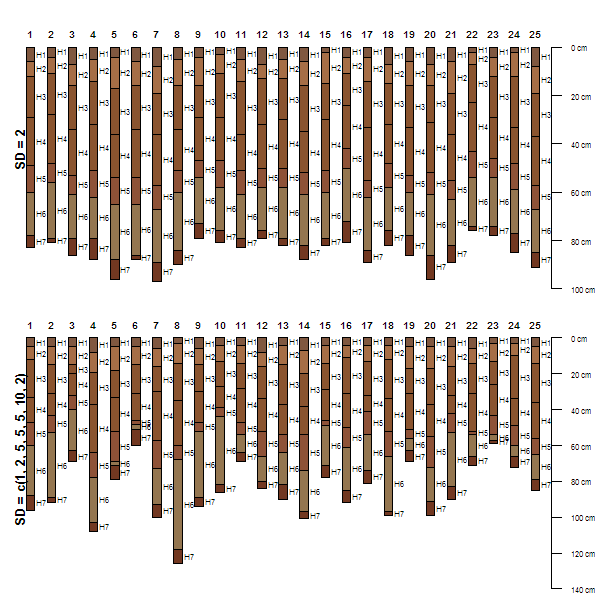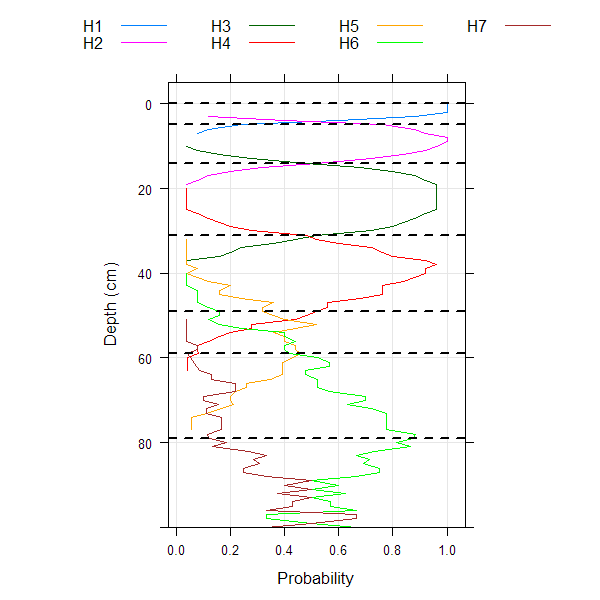sim {aqp} R Documentation

## Simulate Soil Profiles

### Description

Simulate a collection of soil profiles based on the horizonation of a single soil profile.

### Usage

```	sim(x, n=1, iterations=25, hz.sd=2, min.thick=2)
```

### Arguments

 `x` a SoilProfileCollection object containing a single profile from which to draw simulated data `n` the number of requested simulations `iterations` sampling iterations used to determine each horizon thickness `hz.sd` standard deviation used to simulate horizon thickness, can be a vector but must divide evenly into the number of horizons found in `x` `min.thick` minumum horizon thickness allowed in simulation results

### Details

This function generates a collection of simulated soil profiles based on the horizon thickness data associated with a single "template" profile. Simulation is based on sampling from a family of gaussian distribution with means defined by the "template" profile and standard deviation defined by the user.

### Value

A SoilProfileCollection object with `n` simulated profiles, each containing the same number of horizons and same data as `x`.

### Author(s)

D. E. Beaudette

`random_profile`

### Examples

``````# load sample data and convert into SoilProfileCollection
data(sp3)
depths(sp3) <- id ~ top + bottom

# select a profile to use as the basis for simulation
s <- sp3[3, ]

# reset horizon names
s\$name <- paste('H', seq_along(s\$name), sep='')

# simulate 25 new profiles, using 's' and function defaults
sim.1 <- sim(s, n=25)

# simulate 25 new profiles using 's' and variable SD for each horizon
sim.2 <- sim(s, n=25, hz.sd=c(1, 2, 5, 5, 5, 10, 2))

# plot
par(mfrow=c(2,1), mar=c(0, 0, 0, 0))
plot(sim.1)
mtext('SD = 2', side=2, line=-1.5, font=2, cex=0.75)
plot(sim.2)
mtext('SD = c(1, 2, 5, 5, 5, 10, 2)', side=2, line=-1.5, font=2, cex=0.75)
````````````# aggregate horizonation of simulated data
# note: set class_prob_mode=2 as profiles were not defined to a constant depth
sim.2\$name <- factor(sim.2\$name)
a <- slab(sim.2, ~ name, class_prob_mode=2)

# convert to long format for plotting simplicity
library(reshape)
a.long <- melt(a, id.vars=c('top','bottom'), measure.vars=levels(sim.2\$name))

# plot horizon probabilities derived from simulated data
# dashed lines are the original horizon boundaries
library(lattice)
xyplot(top ~ value, groups=variable, data=a.long, subset=value > 0,
ylim=c(100, -5), type=c('l','g'), asp=1.5,
ylab='Depth (cm)', xlab='Probability',
auto.key=list(columns=4, lines=TRUE, points=FALSE),
panel=function(...) {
panel.xyplot(...)
panel.abline(h=s\$top, lty=2, lwd=2)
})
``````[Package aqp version 1.9.1 Index]